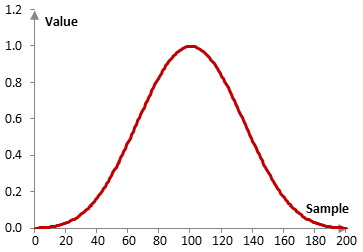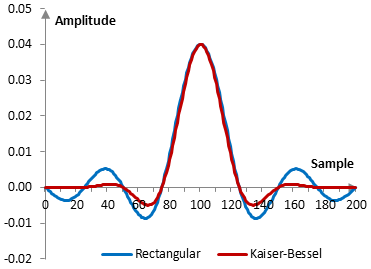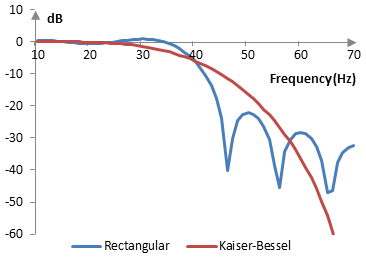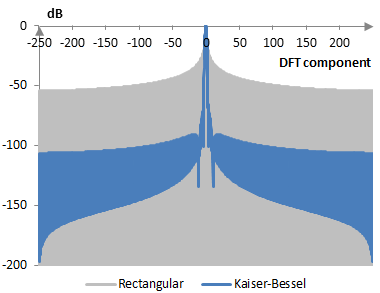# Kaiser-Bessel window

The Kaiser-Bessel window coefficients are given by the following formula

$$a(k)=0.402-0.498 \, \cos(\frac{2\pi k}{N-1})+0.098 \, \cos(\frac{4\pi k}{N-1})-0.001 \, \cos(\frac{6\pi k}{N-1})$$

where N is the length of the filter and k = 0, 1, …, N – 1.

Consider a finite impulse response (FIR) low pass filter of length N = 201. The following is the Kaiser-Bessel window.Given a sampling frequency of 2000 Hz and a filter cutoff frequency of 40 Hz, the impulse response of the filter with a rectangular window (with no window) and with the Kaiser-Bessel window is as follows.The magnitude response of the same filter is shown on the graph below.## Measures for the Kaiser-Bessel window

The following is a comparison of the discrete Fourier transform of the Kaiser-Bessel window and the rectangular window.The Kaiser-Bessel window measures are as follows.

 Coherent gain 0.40 Equivalent noise bandwidth 1.80 Processing gain -2.55 dB Scalloping loss -1.02 dB Worst case processing loss -3.57 dB Highest sidelobe level -65.3 dB Sidelobe falloff -11.8 dB / octave, -39.3 dB / decade Main lobe is -3 dB 1.70 bins Main lobe is -6 dB 2.40 bins Overlap correlation at 50% overlap 0.072 Amplitude flatness at 50% overlap 0.608 Overlap correlation at 75% overlap 0.537 Amplitude flatness at 75% overlap 1.000

Window

### Filtered HTML

• Freelinking helps you easily create HTML links. Links take the form of [[indicator:target|Title]]. By default (no indicator): Click to view a local node.